# 1 推荐模型的分类

## 1.3 矩阵分解

### 1.3.1 显式矩阵分解

user ratings 数据：

Tom, Star Wars, 5
Jane, Titanic, 4
Bill, Batman, 3
Jane, Star Wars, 2
Bill, Titanic, 3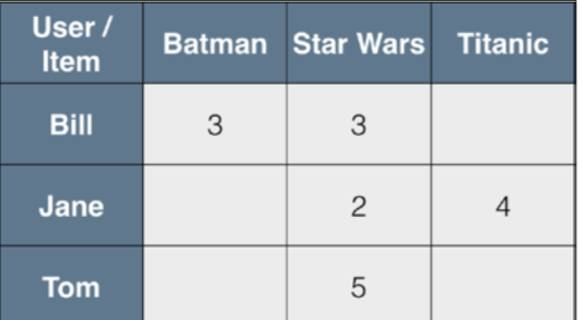MF就是一种直接建模user-item矩阵的方法，利用两个低维度的小矩阵的乘积来表示，属于一种降维的技术。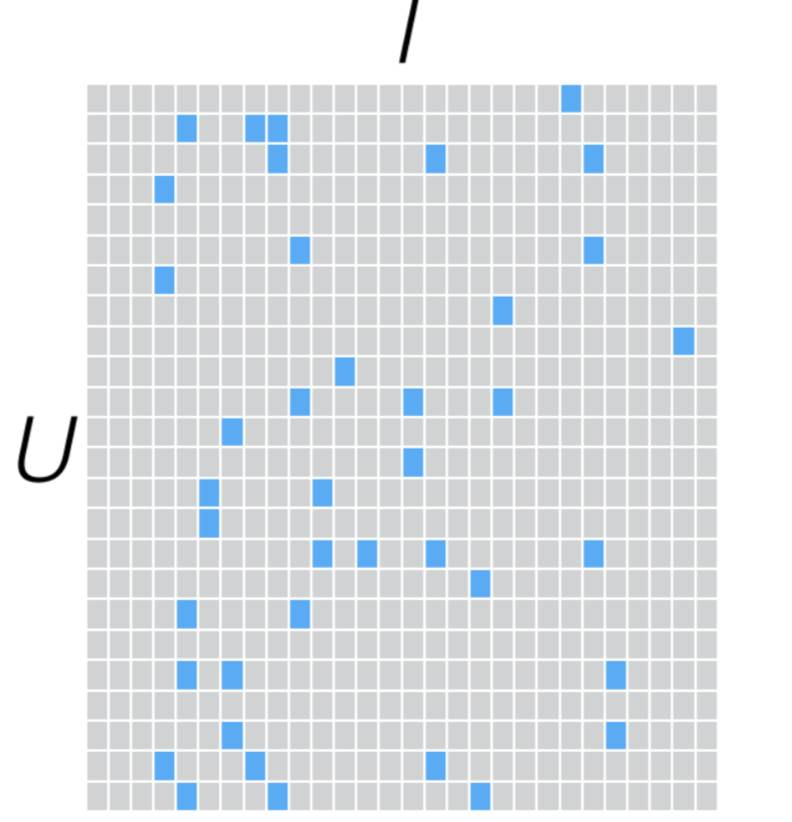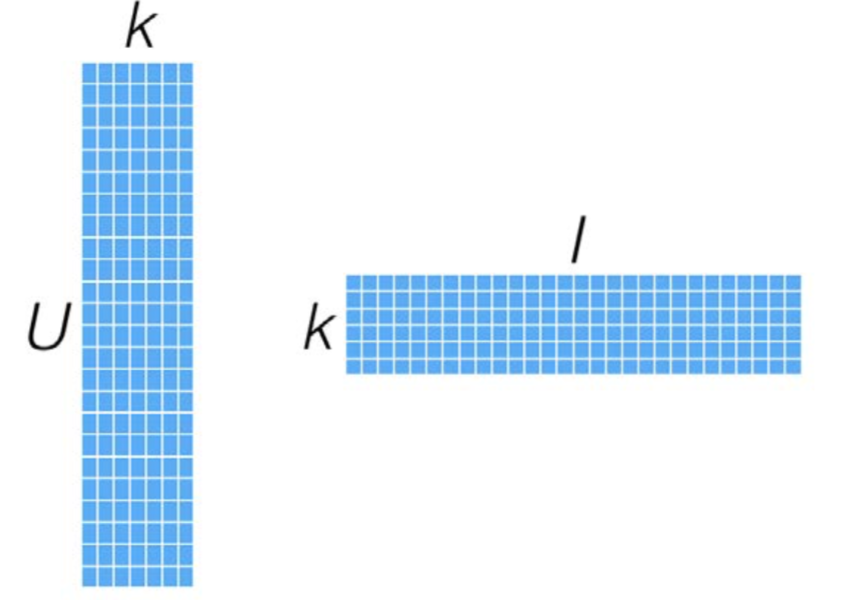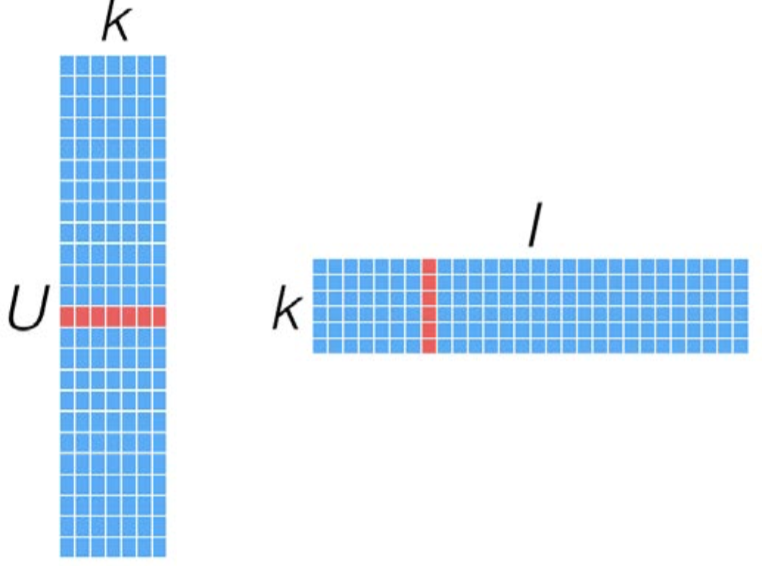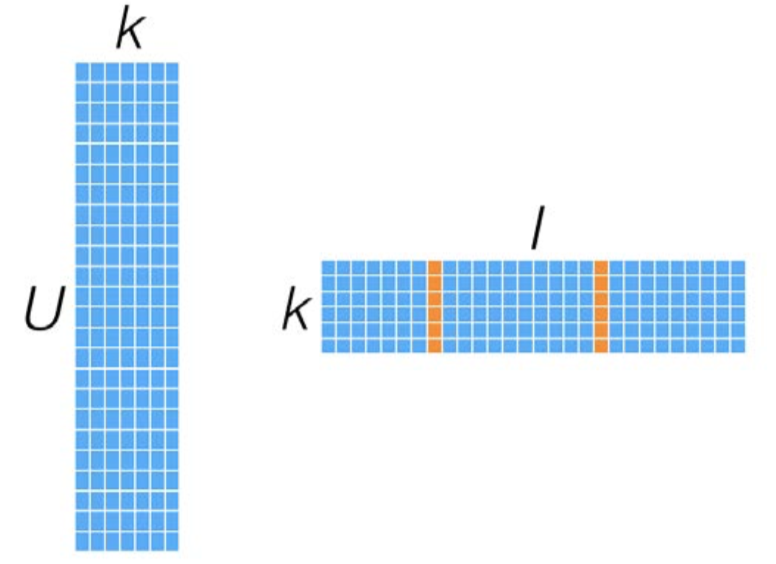### 1.3.2隐式矩阵分解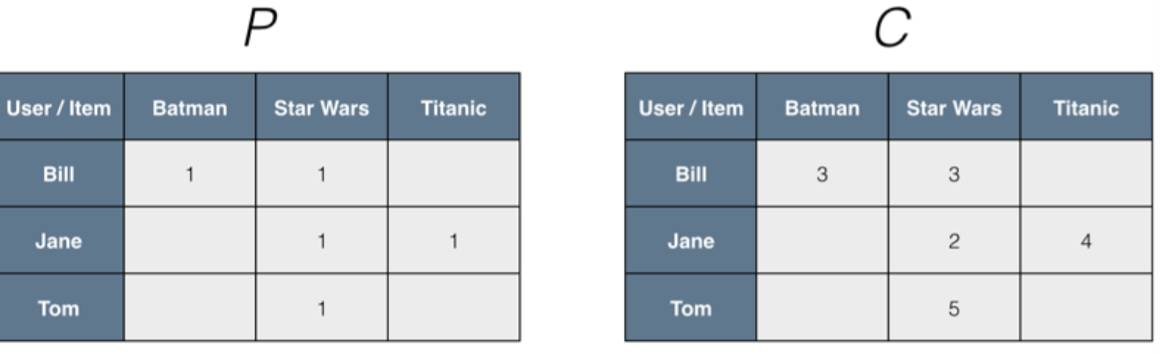### 1.3.3 最小二乘法

ALS的实现原理是迭代式求解一系列最小二乘回归问题。在每一次迭代时,固定用户因子矩阵或是物品因子矩阵中的一个,然后用固定的这个矩阵以及评级数据来更新另一个矩阵。之后,被更新的矩阵被固定住,再更新另外一个矩阵。如此迭代,直到模型收敛(或是迭代了预设好的次数)。

# 2 提取有效特征

rawData = sc.textFile("/Users/youwei.tan/ml-100k/u.data")
print rawData.first()
rawRatings = rawData.map(lambda x: x.split('\t'))
rawRatings.take(5)
#数据分别是userId，itemId，rating和timestamp

196	242	3	881250949

[[u'196', u'242', u'3', u'881250949'],
[u'186', u'302', u'3', u'891717742'],
[u'22', u'377', u'1', u'878887116'],
[u'244', u'51', u'2', u'880606923'],
[u'166', u'346', u'1', u'886397596']]

from pyspark.mllib.recommendation import Rating, ALS
ratings = rawRatings.map(lambda x: Rating(int(x),int(x),float(x)))
print ratings.take(5)

[Rating(user=196, product=242, rating=3.0),
Rating(user=186, product=302, rating=3.0),
Rating(user=22, product=377, rating=1.0),
Rating(user=244, product=51, rating=2.0),
Rating(user=166, product=346, rating=1.0)]


# 3 训练推荐模型

rank:对应ALS模型中的因子个数,也就是在低阶近似矩阵中的隐含特征个数。因子个数一般越多越好。但它也会直接影响模型训练和保存时所需的内存开销,尤其是在用户和物品很多的时候。因此实践中该参数常作为训练效果与系统开销之间的调节参数。通常,其合理取值为10200

iterations:对应运行时的迭代次数。ALS能确保每次迭代都能降低评级矩阵的重建误差,但一般经少数次迭代后ALS模型便已能收敛为一个比较合理的好模型。这样,大部分情况下都没必要迭代太多次(10次左右一般就挺好)。

lambda:该参数控制模型的正则化过程,从而控制模型的过拟合情况。其值越高,正则化越严厉。该参数的赋值与实际数据的大小、特征和稀疏程度有关。和其他的机器学习模型一样,正则参数应该通过用非样本的测试数据进行交叉验证来调整。



model = ALS.train(ratings, 50, 10, 0.01)
userFeatures = model.userFeatures()
print userFeatures.take(2)

输出结果：[(4, array('d', [-0.4886532723903656, -0.5069043636322021, -0.25953221321105957, 0.28663870692253113,
-0.23391617834568024, -0.1597181111574173, -0.6053574085235596, -0.05088397115468979, -0.13468056917190552,
-0.06684212386608124, -0.1647985279560089, -0.3268186151981354, 0.04303080216050148, -0.37185072898864746,
-0.07605815678834915, -0.18150471150875092, 0.4730634391307831, -0.17112521827220917, 0.011302260681986809,
0.025912854820489883, 0.22327645123004913, -0.553532063961029, -0.19743777811527252, -0.06508021801710129,
0.11410114169120789, -0.2178303301334381, -0.12165536731481552, 0.1517478972673416, -0.41739341616630554,
-0.3790307939052582, 0.09140809625387192, 0.438218355178833, -0.40334436297416687, -0.26500797271728516,
-0.17151066660881042, -0.056731898337602615, 0.014920198358595371, -0.4036138951778412, -0.503244161605835,
-0.35094475746154785, -0.2583906650543213, 0.15083833038806915, -0.02699241414666176, -0.5867881774902344,
0.6203442215919495, -0.5354999303817749, 0.49261313676834106, 0.36879995465278625, 0.217197448015213,
0.2137724757194519])),
(8, array('d', [-0.3435080349445343, -0.23504899442195892, 0.01265001017600298, 0.37398409843444824,
-0.12563304603099823, 0.35209083557128906, 0.14092524349689484, -0.03852889686822891, 0.35797831416130066,
-0.21024121344089508, -0.4891291558742523, 0.13990764319896698, -0.2572784125804901, 0.7792724370956421,
0.2034142017364502, 0.5507923364639282, -0.047871965914964676, 0.24563290178775787, 0.14227859675884247,
-0.3111373484134674, 0.6031113266944885, -0.5081701874732971, 0.39406323432922363, 0.17398110032081604,
0.7309723496437073, 0.38500913977622986, -0.39698582887649536, 0.3925837576389313, -0.14110979437828064,
-0.3914717435836792, 0.36928772926330566, 0.6285825967788696, -0.574873149394989, -0.4255753457546234,
-0.14399465918540955, -0.11449692398309708, 0.32894405722618103, 0.17887713015079498, 0.23037177324295044,
-0.17776504158973694, -0.17060403525829315, -0.28235891461372375, -0.33164700865745544, -0.05743246525526047,
0.0831192284822464, -0.007868407294154167, 0.0913839191198349, 0.6946220993995667, 0.3273734152317047,
-0.22331389784812927]))]

注意,MLlib中ALS的实现里所用的操作都是延迟性的转换操作。所以,只在当用户因子或物品因子结果RDD调用了执行操作时,实际的计算才会发生。要强制计算
发生,则可调用Spark的执行操作,如count。





print model.userFeatures().count()
print model.productFeatures().count()

输出结果：943
1682

MLlib中标准的矩阵分解模型用于显式评级数据的处理。若要处理隐式数据,则可使用trainImplicit函数。其调用方式和标准的train模式类似,但多了一个可设置的alpha参数也是一个正则化参数,lambda应通过测试和交叉验证法来设置

alpha 参数指定了信心权重所应达到的基准线。该值越高则所训练出的模型越认为用户与他所没评级过的电影之间没有相关性。

# 4 使用推荐模型

## 4.1用户推荐

MovieLens 100k数据集生成电影推荐

MLlib的推荐模型基于矩阵分解,因此可用模型所求得的因子矩阵来计算用户对物品的预计评级。下面只针对利用MovieLens中显式数据做推荐的情形,使用隐式模型时的方法与之相同。



print len(userFeatures.first())
predictRating = model.predict(789,123)
print predictRating

输出结果：50
3.18324449604

可以看到,该模型预测用户789对电影123的评级为3.18。

predict函数同样可以以(user, item)ID对类型的RDD对象为输入,这时它将为每一对都生成相应的预测得分。我们可以借助这个函数来同时为多个用户和物品
进行预测。

要为某个用户生成前K个推荐物品,可借助MatrixFactorizationModel所提供的recommendProducts函数来实现。该函数需两个输入参数:user和num。其中user
是用户ID,而num是要推荐的物品个数。

返回值为预测得分最高的前num个物品。这些物品的序列按得分排序。该得分为相应的用户因子向量和各个物品因子向量的点积。

现在,计算给用户789推荐的前10个物品，代码如下：




topKRecs = model.recommendProducts(789,10)
print '给用户userId推荐其喜欢的item：'
for rec in topKRecs:
print rec

输出结果：给用户userId推荐其喜欢的item：
Rating(user=789, product=48, rating=5.572495011959803)
Rating(user=789, product=56, rating=5.510520005211698)
Rating(user=789, product=346, rating=5.295860840700527)
Rating(user=789, product=192, rating=5.08681379224634)
Rating(user=789, product=92, rating=5.01867372270688)
Rating(user=789, product=526, rating=5.016016542149785)
Rating(user=789, product=182, rating=4.9996960801921695)
Rating(user=789, product=179, rating=4.9877606036865005)
Rating(user=789, product=100, rating=4.971601345359906)
Rating(user=789, product=9, rating=4.969371624015052)

检验推荐内容

要直观地检验推荐的效果,可以简单比对下用户所评级过的电影和被推荐的那些电影
下面查看用户789评级过的电影，代码如下：

moviesForUser = ratings.groupBy(lambda x : x.user).mapValues(list).lookup(789)
#print ratings.groupBy(lambda x : x.user).mapValues(list).lookup(789)
#print ratings.groupBy(lambda x : x.user).map(lambda (x,y): (x, list(y))).lookup(789)
#print moviesForUser

print '用户对%d部电影进行了评级'%len(moviesForUser)
print '源数据中用户(userId=789)喜欢的电影(item)：'
for i in sorted(moviesForUser,key=lambda x : x.rating,reverse=True):
print i.product

输出结果：用户对33部电影进行了评级

127
475
9
50
150
276
129
100
741
1012
93
293
181
1008
508
1007
124
1161
294
1
284
1017
111
742
248
249
591
288
762
628
137
151
286

movies = sc.textFile("/Users/youwei.tan/ml-100k/u.item")
titles = movies.map(lambda line: (int(line.split('|')),line.split('|'))).collectAsMap()
print titles

输出结果：Toy Story (1995)

for i,rec in enumerate(topKRecs):
print 'rank:'+str(i)+' '+str(titles[rec.product])+':'+str(rec.rating)

输出结果：rank:0 Hoop Dreams (1994):5.57249501196
rank:1 Pulp Fiction (1994):5.51052000521
rank:2 Jackie Brown (1997):5.2958608407
rank:3 Raging Bull (1980):5.08681379225
rank:4 True Romance (1993):5.01867372271
rank:5 Ben-Hur (1959):5.01601654215
rank:6 GoodFellas (1990):4.99969608019
rank:7 Clockwork Orange, A (1971):4.98776060369
rank:8 Fargo (1996):4.97160134536
rank:9 Dead Man Walking (1995):4.96937162402

## 4.2物品推荐

MovieLens 100k数据集生成相似电影

MatrixFactorizationModel当前的API不能直接支持物品之间相似度的计算。所以我们要自己实现。这里我们使用余弦相似度来衡量相似度。



import numpy as np
def cosineSImilarity(x,y):
return np.dot(x,y)/(np.linalg.norm(x)*np.linalg.norm(y))
testx = np.array([1.0,2.0,3.0])
print cosineSImilarity(testx,testx)





itemId = 567
itemFactor = model.productFeatures().lookup(itemId)
print itemFactor

#print model.productFeatures().collect()
#print model.productFeatures().take(1)
sims = model.productFeatures().map(lambda (id,factor):(id,cosineSImilarity(np.array(factor),
np.array(itemFactor))))

sims.sortBy(lambda (x,y):y,ascending=False).take(10)



array('d',
[-0.14139963686466217, 0.6053799390792847, -0.4522729516029358, 0.962456226348877, 0.2535054385662079, -0.4816886782646179,
-0.7732354402542114, 0.7280293703079224, -0.018588555976748466, 0.7229052782058716, 0.44682827591896057, 0.315813809633255,
-0.4070151746273041, -0.08554915338754654, -0.2362387776374817, -0.4164128005504608, -0.2916576862335205, 0.8387507200241089,
-0.10055144876241684, -0.5498601198196411, -0.11159903556108475, -1.4316672086715698, -1.1710675954818726, -0.5741096138954163,
0.30021053552627563, -0.5658889412879944, -0.2256290316581726, 1.0022759437561035, 0.4253554940223694, -0.03920847177505493,
-0.2296391874551773, 0.8324244022369385, 0.1434708833694458, 0.1821751743555069, -0.709479570388794, -0.7556734085083008,
0.9100260734558105, -0.4104197025299072, 0.22313174605369568, -0.5352709293365479, -0.07432051002979279, 0.42420247197151184,
-0.10177311301231384, -0.09117455780506134, -0.5774176120758057, 0.13856376707553864, 0.7275233268737793, -0.12974925339221954,
0.7839331030845642, 0.3163391649723053])

[(567, 0.99999999999999989),
(563, 0.7309392456908036),
(1376, 0.72832388806473258),
(413, 0.71275894340962187),
(1291, 0.70333653584495592),
(436, 0.70019468584044475),
(96, 0.69824883927286607),
(746, 0.69715419740193796),
(331, 0.69497368094311607),
(408, 0.69223520237106451)]

# 5 推荐模型效果的评估

## 5.1 均方差



actual = moviesForUser
actualRating = actual.rating
print '用户789对电影1012的实际评级',actualRating
predictedRating = model.predict(789, actual.product)
print '用户789对电影1012的预测评级',predictedRating
squaredError = np.power(actualRating-predictedRating,2)
print '实际评级与预测评级的MSE',squaredError



用户789对电影1012的实际评级 4.0

moviesForUser

输出结果：
[[Rating(user=789, product=1012, rating=4.0),
Rating(user=789, product=127, rating=5.0),
Rating(user=789, product=475, rating=5.0),
Rating(user=789, product=93, rating=4.0),
Rating(user=789, product=1161, rating=3.0),
Rating(user=789, product=286, rating=1.0),
Rating(user=789, product=293, rating=4.0),
Rating(user=789, product=9, rating=5.0),
Rating(user=789, product=50, rating=5.0),
Rating(user=789, product=294, rating=3.0),
Rating(user=789, product=181, rating=4.0),
Rating(user=789, product=1, rating=3.0),
Rating(user=789, product=1008, rating=4.0),
Rating(user=789, product=508, rating=4.0),
Rating(user=789, product=284, rating=3.0),
Rating(user=789, product=1017, rating=3.0),
Rating(user=789, product=137, rating=2.0),
Rating(user=789, product=111, rating=3.0),
Rating(user=789, product=742, rating=3.0),
Rating(user=789, product=248, rating=3.0),
Rating(user=789, product=249, rating=3.0),
Rating(user=789, product=1007, rating=4.0),
Rating(user=789, product=591, rating=3.0),
Rating(user=789, product=150, rating=5.0),
Rating(user=789, product=276, rating=5.0),
Rating(user=789, product=151, rating=2.0),
Rating(user=789, product=129, rating=5.0),
Rating(user=789, product=100, rating=5.0),
Rating(user=789, product=741, rating=5.0),
Rating(user=789, product=288, rating=3.0),
Rating(user=789, product=762, rating=3.0),
Rating(user=789, product=628, rating=3.0),
Rating(user=789, product=124, rating=4.0)]]



userProducts = ratings.map(lambda rating:(rating.user,rating.product))
print '实际的评分:',userProducts.take(5)

#预测所有用户对电影的相应评分
print model.predictAll(userProducts).collect()
predictions = model.predictAll(userProducts).map(lambda rating:((rating.user,rating.product), rating.rating))
print '预测的评分:',predictions.take(5)

ratingsAndPredictions = ratings.map(lambda rating:((rating.user,rating.product),rating.rating)).join(predictions)
print '组合预测的评分和实际的评分:',ratingsAndPredictions.take(5)

MSE = ratingsAndPredictions.map(lambda ((x,y),(m,n)):np.power(m-n,2)).reduce(lambda x,y:x+y)/ratingsAndPredictions.count()
print '模型的均方误差:',MSE
print '模型的均方根误差:',np.sqrt(MSE)



实际的评分: [(196, 242), (186, 302), (22, 377), (244, 51), (166, 346)]
Rating(user=316, product=1084, rating=4.01303094210043)

((541, 1084), 4.087339438976559), ((181, 1084), 2.0708407464846563)]

((472, 584), (1.0, 1.5594002961174487)), ((752, 316), (3.0, 2.822048986057712)), ((18, 428), (3.0, 3.190997207172113))]

## 5.2 K值平均准确率

K值平均准确率(MAP)的意思是整个数据集上的K值平均准确率(Average Precision at Kmetric,MAP)的均值。APK是信息检索中常用的一个指标。它用于衡量针对某个查询所返回的“前K个”文档的平均相关性。对于每次查询,我们会将结果中的前K个与实际相关的文档进行比较。

MAP指标计算时,结果中文档的排名十分重要。如果结果中文档的实际相关性越高且排名也更靠前,那APK分值也就越高。由此,它也很适合评估推荐的好坏。因为推荐系统也会计算“前K个”推荐物,然后呈现给用户。如果在预测结果中得分更高(在推荐列表中排名也更靠前)的物品实际上也与用户更相关,那自然这个模型就更好。MAP和其他基于排名的指标同样也更适合评估隐式数据集上的推荐。这里用MSE相对就不那么合适。



def avgPrecisionK(actual, predicted, k):
if len(predicted) > k:
predK = predicted[:k]
else:
predK = predicted
score = 0.0
numHits = 0.0
for i,p in enumerate(predK):
if p in actual and p not in predK:
numHits = numHits + 1
score = score + numHits/(i+1)
if not actual:
return 1.0
else:
return score/min(len(actual),k)





actualMovies = [rating.product for rating in moviesForUser]
predictMovies = [rating.product for rating in topKRecs]
print '实际的电影：',actualMovies
print '预测的电影：',predictMovies



实际的电影： [1012, 127, 475, 93, 1161, 286, 293, 9, 50, 294, 181, 1, 1008, 508, 284, 1017, 137, 111, 742, 248, 249, 1007, 591,
150, 276, 151, 129, 100, 741, 288, 762, 628, 124]



MAP10 = avgPrecisionK(actualMovies,predictMovies,10)
print MAP10



0.0



itemFactors = model.productFeatures().map(lambda (id,factor):factor).collect()
itemMatrix = np.array(itemFactors)
print itemMatrix
print itemMatrix.shape



[ 0.02446744 -1.29959726 -0.41932318 ...,  0.81818616  0.18477531
0.24459557]
[-0.24553037 -1.99444282 -0.25264686 ...,  0.82401907  0.8064388
-0.30488601]
[ 0.0659191  -1.3440671  -1.0006181  ...,  0.98762459  0.80993211
-0.1881171 ]
...,
[ 0.03007785 -0.199938   -0.06636383 ..., -0.0149461   0.06697606
0.00769717]
[-0.17347991 -0.24106073 -0.42270574 ...,  0.33844769 -0.01864041
0.06676605]
[ 0.00804477 -0.12564704 -0.09706354 ..., -0.0166881  -0.18410273
-0.16378592]]
(1682, 50)




#便于后面进行map计算的时候使所有map都有效，这里在实际当中的意思是把数据广播到任何一个节点





userVector = model.userFeatures().map(lambda (userId,array):(userId,np.array(array)))
#print userVector.collect().shape
#print userVector.collect().shape
userVectorId = userVector.map(lambda (userId,x) : (userId,[(xx,i) for i,xx in enumerate(x.tolist())]))
#print userVectorId.collect()
sortUserVectorId = userVectorId.map(lambda (userId,x):(userId,sorted(x,key=lambda x:x,reverse=True)))
sortUserVectorRecId = sortUserVectorId.map(lambda (userId,x): (userId,[xx for xx in x]))
#print sortUserVectorRecId.take(2)
sortUserVectorRecId.count()



943



userMovies = ratings.map(lambda rating: (rating.user,rating.product)).groupBy(lambda (x,y):x)
print userMovies.take(3)
userMovies = userMovies.map(lambda (userId,x):(userId, [xx for xx in x] ))
#print userMovies.take(1)
#allAPK=sortUserVectorRecId.join(userMovies).map(lambda (userId,(predicted, actual)):(userId,avgPrecisionK(actual,predicted,10)))
#print allAPK.map(lambda (x,y):y).reduce(lambda x,y:x+y)/sortUserVectorRecId.count()
allAPK=sortUserVectorRecId.join(userMovies).map(lambda (userId,(predicted, actual)):avgPrecisionK(actual,predicted,2000))
# print allAPK.take(10)
print allAPK.reduce(lambda x,y:x+y)/allAPK.count()



[(2, <pyspark.resultiterable.ResultIterable object at 0x1163fa750>),
(4, <pyspark.resultiterable.ResultIterable object at 0x1163fae90>),
(6, <pyspark.resultiterable.ResultIterable object at 0x1163fa810>)]
0.0

## 5.3 使用MLlib内置的评估函数

RMSEMSE



from pyspark.mllib.evaluation import RegressionMetrics
from pyspark.mllib.evaluation import RankingMetrics
predictedAndTrue = ratingsAndPredictions.map(lambda ((userId,product),(predicted, actual))
:(predicted,actual))
print predictedAndTrue.take(5)
regressionMetrics = RegressionMetrics(predictedAndTrue)
print "均方误差 = %f"%regressionMetrics.meanSquaredError
print "均方根误差 = %f"% regressionMetrics.rootMeanSquaredError



[(5.0, 5.068769168364991), (3.0, 3.078863587357608), (1.0, 1.5594002961174487), (3.0, 2.822048986057712), (3.0, 3.190997207172113)]

MAP

RankingMetrics中的K值平均准确率函数的实现与我们的有所不同,因而结果会不同。但全局平均准确率(Mean Average Precision,MAP,并不设定阈值K)会和当K值较大(比如设为总的物品数目)时我们模型的计算结果相同。



sortedLabels = sortUserVectorRecId.join(userMovies).map(lambda (userId,(predicted, actual)):(predicted,actual))
# print sortedLabels.take(1)
rankMetrics = RankingMetrics(sortedLabels)
print "Mean Average Precision = %f" % rankMetrics.meanAveragePrecision
print "Mean Average Precision(at K=10) = %f" % rankMetrics.precisionAt(10)



Mean Average Precision = 0.072366
Mean Average Precision(at K=10) = 0.069883

# 6 小结

11-2606-15109
08-23118
11-121万+
11-256823
06-16215
02-263046